# Cartesian componentsPage 1

#### WATCH ALL SLIDES

Slide 1CARTESIAN COMPONENTS OF VECTORS

Slide 2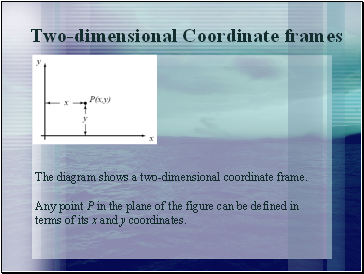## Two-dimensional Coordinate frames

The diagram shows a two-dimensional coordinate frame.

Any point P in the plane of the figure can be defined in terms of its x and y coordinates.

Slide 3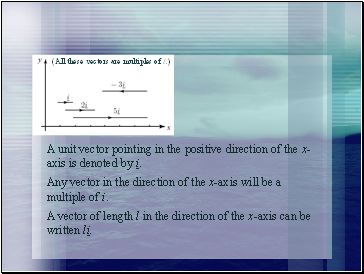A unit vector pointing in the positive direction of the x-axis is denoted by i.

Any vector in the direction of the x-axis will be a multiple of i.

A vector of length l in the direction of the x-axis can be written li.

(All these vectors are multiples of i.)

Slide 4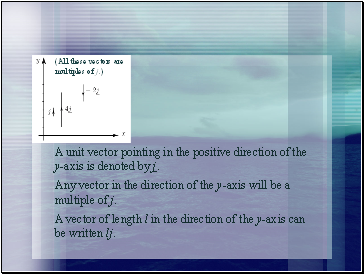(All these vectors are

multiples of j.)

A unit vector pointing in the positive direction of the y-axis is denoted by j.

Any vector in the direction of the y-axis will be a multiple of j.

A vector of length l in the direction of the y-axis can be written lj.

Slide 5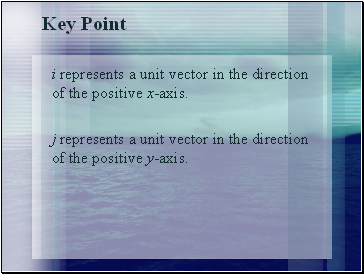## Key Point

i represents a unit vector in the direction of the positive x-axis.

j represents a unit vector in the direction of the positive y-axis.

Slide 6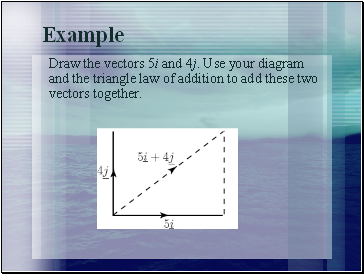Example

Draw the vectors 5i and 4j. Use your diagram and the triangle law of addition to add these two vectors together.

Slide 7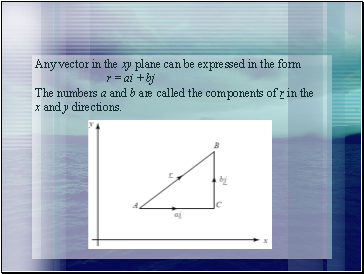Any vector in the xy plane can be expressed in the form

r = ai + bj

The numbers a and b are called the components of r in the

x and y directions.

Slide 8a) Draw an xy plane and show the vectors p = 2i + 3j, and q = 5i + j.

b) Express p and q using column vector notation.

c) Show the sum p + q.

d) Express the resultant p + q in terms of i and j.

Example

Slide 9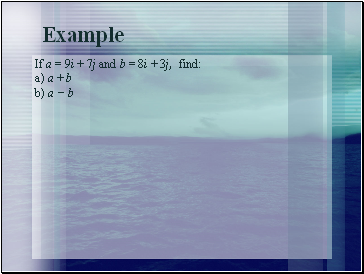If a = 9i + 7j and b = 8i + 3j, find:

a) a + b

b) a − b

Example

Slide 10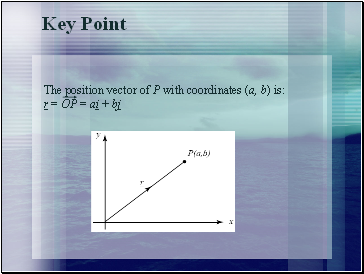Key Point

The position vector of P with coordinates (a, b) is:

r = OP = ai + bj

Slide 11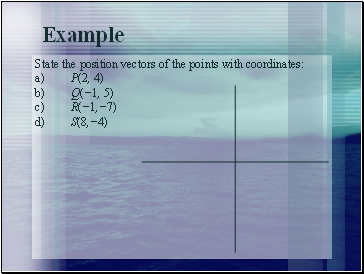State the position vectors of the points with coordinates:

a) P(2, 4)

b) Q(−1, 5)

c) R(−1,−7)

d) S(8,−4)

Example

Slide 12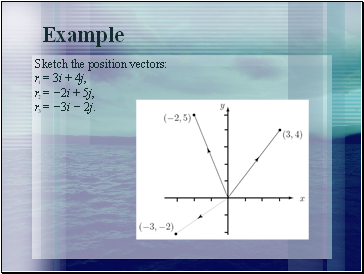Go to page:
1  2